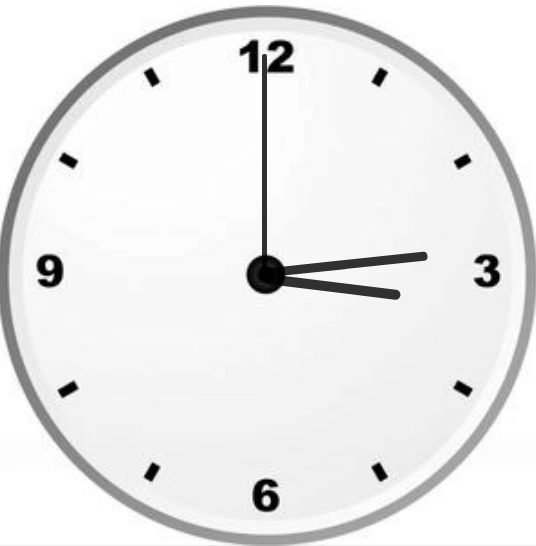# Create an Analog Clock using HTML, CSS and JavaScript

• Difficulty Level : Medium
• Last Updated : 12 Mar, 2021

We are going to build a real-time analog clock using HTML, CSS, and JavaScript.

Prerequisite:

Hey geek! The constant emerging technologies in the world of web development always keeps the excitement for this subject through the roof. But before you tackle the big projects, we suggest you start by learning the basics. Kickstart your web development journey by learning JS concepts with our JavaScript Course. Now at it's lowest price ever!

Approach: We will create three files (HTML file, a CSS file, and JavaScript File), we also have an image of the clock that will be used in the background, and on top of that, we will make an hour, minute, and second hand (using HTML and CSS). These hands will rotate as per the system time (we will use the predefined Date function of JavaScript to calculate the degree of rotations of each hand).

• HTML: It is a simple file having the basic structure of the webpage and ID for the clock’s body and for the second, minute, hour hands.
• CSS: The CSS is used just for making the clock actually look a bit nicer. We have basically centered our clock in the middle of the webpage.
• JavaScript: The JavaScript file will provide the logic behind the rotation of the hands.

Example:

• First we have selected the hour, minute, and second from HTML.
• To get the current time we have used the Date() object provided by the JavaScript. This will give the current seconds, minutes, and hours respectively.
• Now, we have got our hour, minute, and second, and we know that the clock rotates 360 degrees. So, we will convert to convert the rotation of the hands of the clock into degrees. The degree calculation is based on a simple unary method.

## index.html

 ```<``html` `lang``=``"en"``>``<``head``>``    ``<``link` `rel``=``"stylesheet"` `href``=``"style.css"``>``    ``<``script` `src``=``"index.js"``>````<``body``>``    ``<``div` `id``=``"clockContainer"``>``        ``<``div` `id``=``"hour"``>``        ``<``div` `id``=``"minute"``>``        ``<``div` `id``=``"second"``>``    ```````

## style.css

 `#clockContainer {``    ``position``: ``relative``;``    ``margin``: ``auto``;``    ``height``: ``40``vw;``    ``/*to make the height and width responsive*/``    ``width``: ``40``vw;``    ``background``: ``url``(clock.png) ``no-repeat``;``    ``/*setting our background image*/``    ``background-``size``: ``100%``;``}`` ` `#hour,``#minute,``#second {``    ``position``: ``absolute``;``    ``background``: ``black``;``    ``border-radius: ``10px``;``    ``transform-origin: ``bottom``;``}`` ` `#hour {``    ``width``: ``1.8%``;``    ``height``: ``25%``;``    ``top``: ``25%``;``    ``left``: ``48.85%``;``    ``opacity: ``0.8``;``}`` ` `#minute {``    ``width``: ``1.6%``;``    ``height``: ``30%``;``    ``top``: ``19%``;``    ``left``: ``48.9%``;``    ``opacity: ``0.8``;``}`` ` `#second {``    ``width``: ``1%``;``    ``height``: ``40%``;``    ``top``: ``9%``;``    ``left``: ``49.25%``;``    ``opacity: ``0.8``;``}`

## index,js

 `setInterval(() => {``    ``d = ``new` `Date(); ``//object of date()``    ``hr = d.getHours();``    ``min = d.getMinutes();``    ``sec = d.getSeconds();``    ``hr_rotation = 30 * hr + min / 2; ``//converting current time``    ``min_rotation = 6 * min;``    ``sec_rotation = 6 * sec;`` ` `    ``hour.style.transform = `rotate(\${hr_rotation}deg)`;``    ``minute.style.transform = `rotate(\${min_rotation}deg)`;``    ``second.style.transform = `rotate(\${sec_rotation}deg)`;``}, 1000);`

Output:My Personal Notes arrow_drop_up